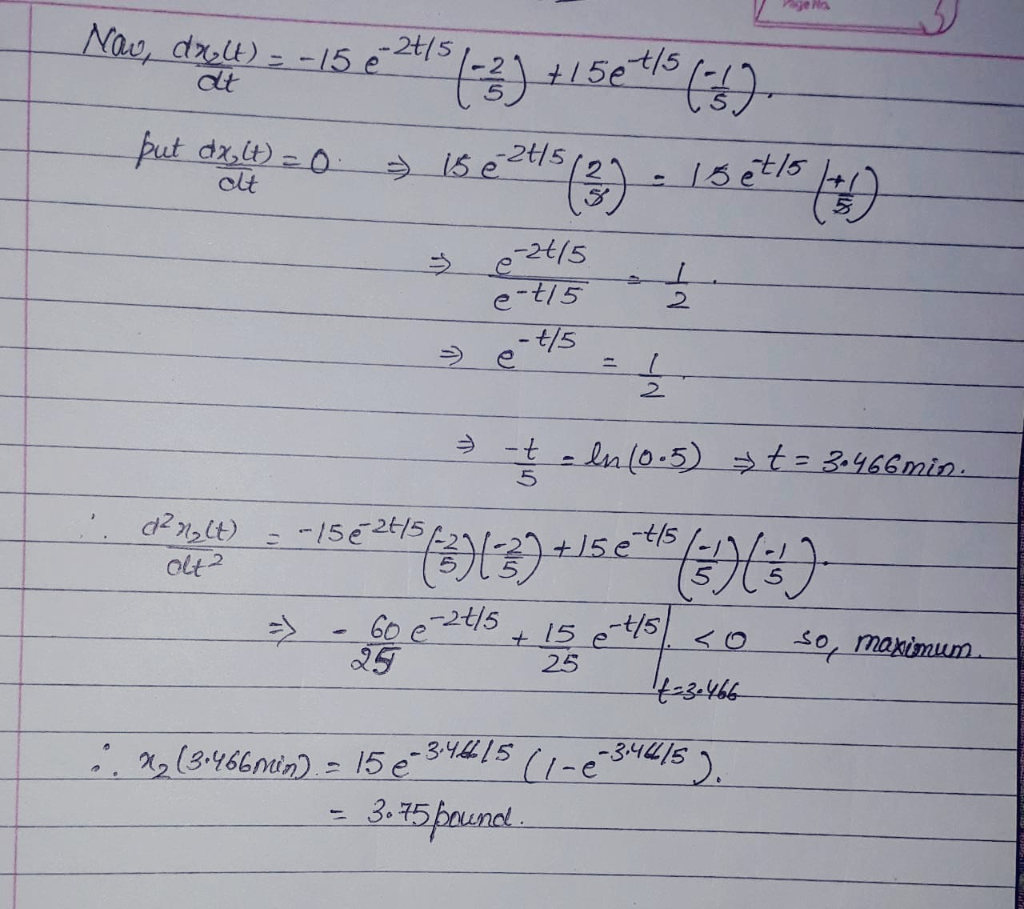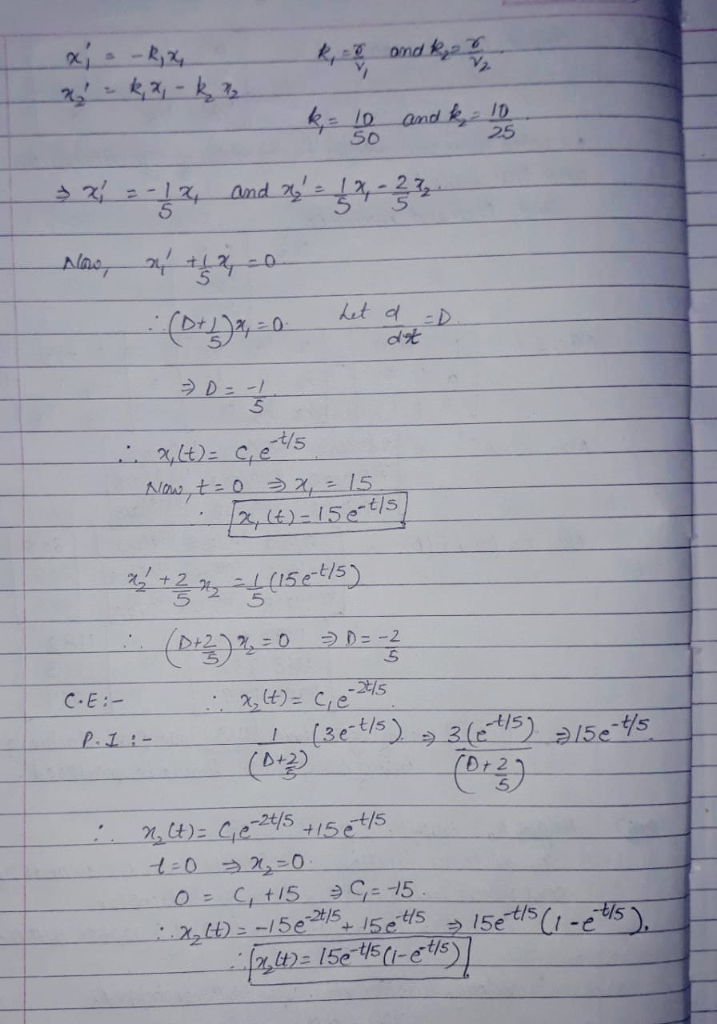Homework Help Question & Answers

1. Consider the following figure below that shows two brine tanks r galmin fresh water Tank 1 ank...1. Consider the following figure below that shows two brine tanks r galmin fresh water Tank 1 anks 2 containing and V, gallons of brine respectively. Fresh water flows into tank 1, while mixed brine flows from tank l into tank 2· Let x(1) denote the amount (in pounds) ofsalt in tank i at time for i-1,2. Ifeach flow rate is r gallons per minute, then a simple account of salt concentration yields the first-order system where 1,2 If V-50, V-25, -10(gal /min), and the initial amounts of salt in the two brine tanks, in pounds, are (0)-15, x(0)-0 a) Find the amount of salt in each tank at time 20 b) Find the maximum amount of salt ever in tank 2Add Answer of: 1. Consider the following figure below that shows two brine tanks r galmin fresh water Tank 1 ank...
More Homework Help Questions Additional questions in this topic.

• a tank is full of water. Find the work recquired to pump the water out of different tank shapes. Use the fact that water weighs 62.5 lf/ft^3 2.(a) Spherical tank full of water (b) Spherical tank i...

Need Online Homework Help?

Get FREE EXPERT Answers
WITHIN MINUTES
Related Questions